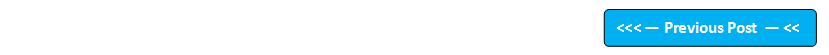## Blog Pages

### 256. Object ArraysPre-requisite -

Assuming that you know the Object Class and AutoBoxing as mentioned in pre-requisites for understanding this post, I am going to explain the Object Arrays concept which allows us to assign the values of any primitive data type to the objects of an Object Class as shown in the below example -

Example -

Object obj[ ] = new Object;

obj = 123;
obj =  'a';
obj = 12.3F;
obj = 12L;
obj = 123.456;
obj = true;

As Object Class is the parent class of all the Classes in Java, All the Wrapper Class i.e. Integer, Byte, Short, Long, Float, Double, Character, Boolean are the child classes of Object Class. Hence we can use Object class in AutoBoxing in order to assign the values of different primitive data types as shown in the above example.

Lets implement this on Eclipse IDE -

1. Launch Eclipse IDE, create a new Java Project 'Project 49' as shown below -

2. As I am going to use AutoBoxing concept in my program, I will change the compiler compliance level to the latest level in my Project -> Properties dialog as shown below -

3. Now create a new Java Class 'ObjectArraysDemo.java' with main( ) method as shown below -

4. Create an Object Class Array, by using the Array Concept as shown below -

5. As the above Object Array can store 5 values, lets assign values of different primitive data types (i.e. int , float, long , char, boolean) -

6. Write the code for printing all the values of different primitive data types using the object elements of Object Array as shown below -

7. Save & Run the Java Class 'ObjectArraysDemo.java' and observe that the values of different primitive data types got printed using the object elements of the Object Array as shown below -

Hence we can use Object Arrays to assign different primitive data type values to the elements of a single Array.

As Number Class is the parent class of the Wrapper Classes Integer, Byte, Short, Long, Float and Double. You can also create Array of objects for Number Class and assign int, byte, short, long, float & double primitive data type values to the same array elements as shown in the below example -

Example -

Number obj[ ] = new Number;

obj = 123;
obj = 123.456;
obj = 123456L;
obj = 12.3F;

But you cannot assign the char or boolean primitive data type values to the object elements of the Number Class type Array.

obj = 'a'; //invalid as the Character class is not a child class of  Number Class.
obj = true;  //invalid as the Boolean class is not a child class of Number Class.

Hence you can use Object Arrays to assign any primitive data type values to the object array elements of the single Object Class Array. And you can use Number Class Arrays to assign numeric primitive data types to the object elements of the Number Class array. Implement the Number Class Array as I have implemented the Object Class Array(I am not implementing it to save my time :) ).